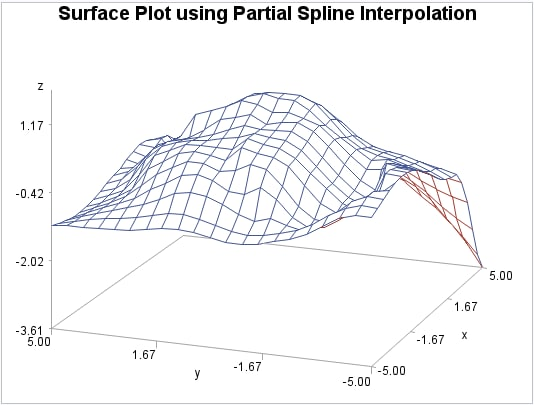# Example 3: Partial Spline Interpolation

 Features: GRID statement options NEAR and PARTIAL Data set: NUMS Sample library member: GTGPART
This example specifies a partial spline interpolation on the GRID statement, using the eight nearest neighbors for computing the estimates of the first, and second derivatives. The output data set, when used in PROC G3D, generates a more smooth surface plot than the surface plot that results from the default interpolation shown in Using the Default Interpolation Method, but does not generate the smoothness of the surface plot that results from the spline interpolation shown in Spline and Smoothing Interpolations.
Surface Plot using Partial Spline Interpolation (gtgpart)## Program

`goptions reset=all border;`
```proc g3grid data=nums out=partial;
grid y*x=z / partial
near=8
axis1=-5 to 5 by .5
axis2=-5 to 5 by .5;
run;```
```title "Surface Plot using Partial Spline
Interpolation";```
```proc g3d data=partial;
plot y*x=z;
run;
quit;```

## Program Description

Set the graphics environment.
`goptions reset=all border;`
Process data with PROC G3GRID. The PARTIAL option specifies that a spline be used to estimate the derivatives for the biquintic polynomial interpolation. The NEAR= option specifies the number of nearest neighbors to be used for computing the estimates of the first, and the second derivatives.
```proc g3grid data=nums out=partial;
grid y*x=z / partial
near=8
axis1=-5 to 5 by .5
axis2=-5 to 5 by .5;
run;```
Define title for the plot.
```title "Surface Plot using Partial Spline
Interpolation";```
Generate the surface plot.
```proc g3d data=partial;
plot y*x=z;
run;
quit;```